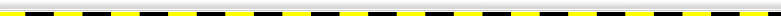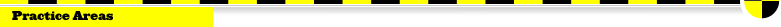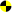Crash ReconstructionTruck Crash ReconstructionMechanical Failure AnalysisTruck Safety & ComplianceTruck Driving StandardsTruck Maintenance AnalysisRunaway Truck CrashesBrake Failure AnalysisAir Brake Failure AnalysisWheel & Hub Failure AnalysisTire Failure AnalysisTruck Underride CollisionsRoadway Safety AnalysisAuto Event Data RecordersTruck Event Data RecordersForensic MappingComputer AnimationDiminished Value InspectionsExpert Testimony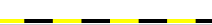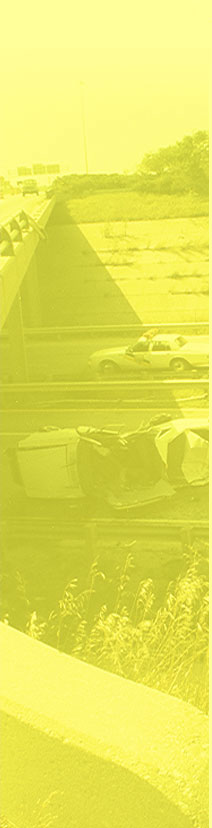Calculating Critical Speed - A Motor-Vehicle Crash Reconstruction Method Fraught with Error John C. Glennon, D.Engr., P.E. August 2006 (copyright) Introduction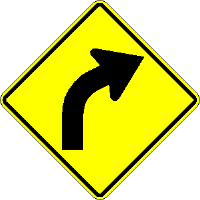The term critical speed as typically used in motor-vehicle crash reconstruction refers to using the centripetal acceleration equation to calculate that speed at which a vehicle will allegedly lose control as a function of cornering radius, tire-pavement friction, and cross-slope. Two common analyses of critical speed are applied as follows To calculate how fast a vehicle was traveling when it lost control rounding a horizontal roadway curve, given the radius, cross slope, and tire-pavement friction.   To calculate the loss-of-control speed for a yawing vehicle based on the measured radius of the yaw marks, the pavement cross slope, and the tire-pavement friction. As commonly applied, both of these methods tend to give inflated speeds. The following discussion first provides a basic primer on the dynamics of a vehicle rounding a circular radius and then points out the common errors associated with each of the two applications. Basic Physics on Vehicle Cornering Sir Isaac Newton tells us that a body moving in a straight line will continue in a straight line unless acted on by an external force. A body moving on a circular path with constant speed will have a changing velocity (directional speed) due to the body's changing direction. This velocity change with time, called centripedal acceleration, has a radial direction toward the center of the circular movement and is given by the following equation: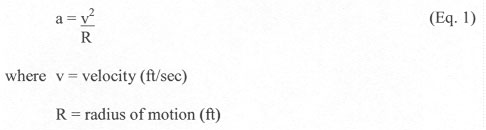Since Newton's second law tells us that a force has to act on the body to produce an acceleration, the following is true: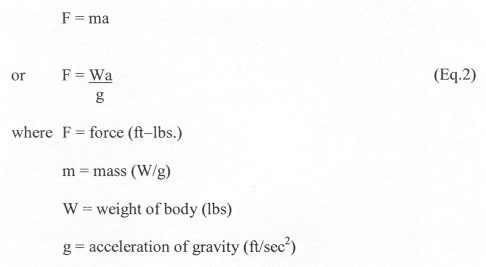substituting Equation 1 into Equation 2, the following can be written: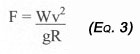The lateral force on a vehicle moving in a circular motion on a pavement surface is produced by the frictional force between the tires and the roadway as follows: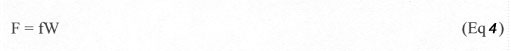where f = the friction demand between the tire and roadway Substituting Equation 4 into Equation 3 yields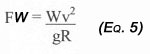Converting Equation 5 to allow for the velocity, S, in mph, and accounting for the roadway curve superelevation, e, yields the familiar form of the centripedal acceleration equation.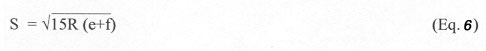The Critical Speed for a Horizontal Roadway Curve Critical speed used in this context is a term for the speed at which a vehicle will lose lateral control on a given roadway curve. The normally flawed procedure is to assume the highway curve radius as the critical path taken by the vehicle. On the surface, this procedure appears reasonable since the driver is apparently attempting to drive around the roadway curve. This theory fails, however, when it's used to compute the speed at loss of control on a dry surface with a reasonably good tire-pavement friction value of, say 0.70. The procedure assumes that the driver exactly followed the roadway curve radius with a steady-state (not braking or speeding up) lateral acceleration (commonly called centrifugal force) of 0.7 g's. Studies consistently show, however, both that drivers' normally steer instantaneous path radii that are sharper than the roadway curve and also that drivers cannot tolerate steady-state lateral accelerations greater than about 0.30 g's. Therefore, a more likely scenario is that the driver, because of inattention or surprise, proceeded into the roadway curve without any initial steering and then needed to turn a sharper path than the roadway curve to stay on the roadway, thereby generating a temporal lateral acceleration greater than 0.7 g's at a speed much lower than the speed calculated by assuming the roadway curve radius is the vehicle path radius. Another likely scenario is where the driver entered the roadway curve too fast, generating .30–0.35 g's of lateral acceleration. Because the driver could not tolerate this level of lateral acceleration, he tried to compensate by flattening his path and/or braking, either of which could induce loss of control. As an illustration, consider the study by Glennon and Weaver1 that shows that drivers consistently steer sharper radii than the radius of the roadway curve. This study provides the following table: Table 1 Percentiles Of Vehicle Path Versus Roadway Curve Path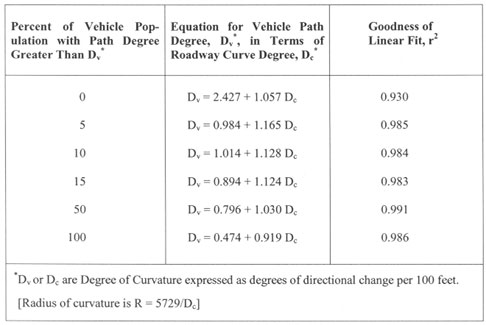If, for example, a ten–percentile driver (only 10% of all drivers use a sharper path) is considered as the criterion, the critical vehicle path on a 8-degree roadway curve can be calculated as 9.92 degrees or a 577–foot path radius on this 716–foot roadway curve radius. Using the results of a study by Rice, et.al 2, which shows that most drivers cannot handle steady-state lateral g levels greater than about 0.30, the critical speed with this lateral acceleration on an 8-degree roadway curve with say 6% superelevation can be calculated as follows:This speed contrasts with the usual practice of using the roadway curve radius of 573 foot, with, say, a tire–pavement coefficient of 0.70, which would give the following calculations: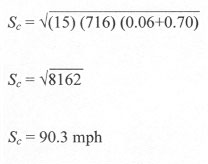As the reader can see, the theoretical critical speed calculation is far greater than the more realistic empirical calculation. The following table shows a series of similar calculations, demonstrating the dramatic difference between the theoretical critical speed and a more realistic critical speed: Table 2 Comparison Of Critical Speed Calculation Methods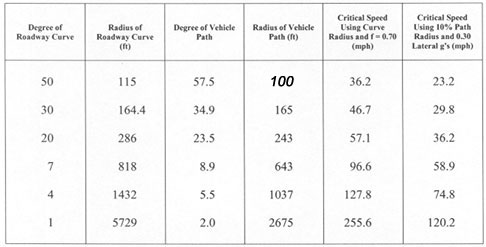In summary, given that all drivers drive a path radius sharper than the roadway curve and given than most drivers cannot tolerate lateral accelerations much greater the 0.3 g's, a more realistic critical speed calculation should take these expected characteristics into account to arrive at an expected loss of control speed. The Myth of Calculating Loss-of-Control Speeds from Yaw Mark Radii Most crash reconstruction books present a method for calculating the loss-of-control speed for an out-of-control vehicle that left yaw marks on the pavement. Yaw marks are tire marks characterized by diagonal striations left by a rotating tire sliding partially sideway on the roadway. Let's be entirely clear that this reconstruction method is presented in several well-used accident reconstruction texts. However, just because the method first showed up in one prominent text and subsequently has been copied by several "me to" texts doesn't make it right. This "critical speed" method must withstand the rigors of scientific inquiry, which it does not. This critical speed method is misused to estimate the initial speed of an out-of-control vehicle. It is erroneously based on the assumption that the steady-state centripedal acceleration equation can be applied using the radius of the yaw marks as input to the equation. This equation can be stated as follow: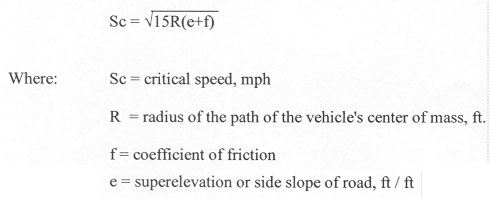In reality, this equation explains the dynamics of a steady-state point mass object and, therefore, should not be applied using tiremarks that are associated with a rotating out-of-control vehicle. The only time yawmark radius can ever be reasonably used to calculate vehicle speed is when a vehicle is steered right at critical slip and deposits yawmarks with the vehicle (marginally) under control. The general procedure for this faulty method has the following steps: Conduct a chord-offset measurement of the yawmark(s).   Calculate the radius of the yawmark(s) from the chord-offset measurements.   Add or subtract half the wheel track to obtain the path radius for the vehicle's center of mass.   Measure or estimate tire-pavement friction.   Measure the roadway cross-slope relative to the yaw mark.   Calculate the loss-of-control speed using the centripetal acceleration equation. The actual path of an out-of-control yawing vehicle can be mathematically described as a spiral. A spiral is a locus of points forming a curved path with continuously decreasing radius. The loss of control point, of course, is usually at or just before the beginning of the tire marks. Starting with the beginning of the yawmark, if a relatively short chord is used, the calculated radius will be relatively high and the calculated speed will be relatively high. If a relatively long chord is used, the calculated radius will be lower and the calculated speed will be lower. Also, as the chord measurement is made starting further along the yaw mark, the calculated radius will be lower and the calculated speed will be lower. Because the chord-offset method is intended to measure a circular curve, which for this application is applied to a spiral curve, because the measurement must be made downstream from the loss-of-control point where the vehicle is now rotating around its center of mass, and because the radius calculated by the chord-offset method is very sensitive to small measurement errors, this method can produce substantial error in estimating loss-of-control speed. The alternative to calculating speed from the centripedal acceleration equation by measuring the radius of yawmark is, of course, to treat the crash as any other single-vehicle crash where the reconstruction considers the dissipation of energy through skidding, rollover, collision with fixed objects, etc. References Glennon, John C., and Weaver, Graeme D., The Relationship of Vehicle Paths to Highway Curve Design, Texas Transportation Institute, Research Report No. 134-5, 1971.   Rice, R.S.,and Dell, Amico F., An Experimental Study od Automobile Driver Characteristics and Capabilities, Calspan Report No. ZS-5208-k-1, March 1974   Glennon, John C., Neuman, Timothy R., and McHenry, Brian G., Prediction of the Sensitivity of Vehicle Dynamics to Highway Curve Geometrics Using Computer Simulation, Transportation Research Record 923, 1983.   Glennon, J. C., Neuman, T. R., and Leisch, J. E., Safety and Operational Considerations for Design of Rural Highway Curves, Federal Highway Administration, 1983.   Neuman, Timothy R., Glennon, John C., and Saag, James B., Accident Analysis for Highway Curves, Transportation Research Record 923, 1983.   Glennon, John C., Effect of Alinement on Highway Safety: A Synthesis of Prior Research, Transportation Research Board, State of the Art Report 6, 1987.   Glennon, John C., and Weaver, Graeme D., Highway Curve Design for Safe Vehicle Operations, Highway Research Record 371, 1972.   Glennon, John C., State of the Art Related to Safety Criteria for Highway Curve Design, Texas Transportation Institute, Research Report No. 134-4, 1969.   Onofrio, John A., and Howarth, Louis E., Forensic Engineering Review of Critical Speed Formula, NAFE Journal, December 2005.   Manning, Lindley, Critical Speed in a Curve – Update, NAFE Journal, june 1990.   Manning, Lindley, Highway Speed vs. Sideslip (Critical Speed in a Curve), NAFE Journal, October 1984. OTHER PAPERSAbout the Author Dr. John C. Glennon is a traffic engineer with over 45 years experience. He has over 120 publications. He is the author of the book "Roadway Safety and Tort Liability" and is frequently called to testify both about roadway defects and as a crash reconstructionist. RESUME OF JOHN C. GLENNONBook , books,pavement edge drop expert, pavement edge drop off expert, guardrail expert, work zone safety expert, construction zone safety expert, roadway hydroplning expert, traffic engineering expert, traffic sign expert, traffic signal expert, pavement marking expert, highway safety expert